## Data Science with Python

Certification Training
20576 Learners
4 Chapters +

# Data Visualization in Python using matplotlib

This is the ‘Data Visualization in Python using matplotlib’ tutorial which is part of the Data Science with Python course offered by Simplilearn. We will learn about Data Visualization and the use of Python as a Data Visualization tool.

## Objectives

In this tutorial, we will be learning

• Explain what data visualization is and its importance in our world today
• Understand why Python is considered one of the best data visualization tools
• Describe matplotlib and its data visualization features in Python
• List the types of plots and the steps involved in creating these plots

## Data Visualization

Data visualization is the technique to present the data in a pictorial or graphical format. It enables stakeholders and decision makers to analyze data visually. The data in a graphical format allows them to identify new trends and patterns easily.

### Example

Let's look at an example below.

We are a sales manager in a leading global organization. The organization plans to study the sales details of each product across all regions and countries. This is to identify the product which has the highest sales in a particular region end up the production.This research will enable the organization to increase the manufacturing of that product in the particular region. The data involved in this research might be huge and complex. The research on this large numeric data is difficult and time-consuming when it is performed manually.

When this numerical data is plotted on a graph for converted to charts it's easy to identify the patterns and predicted the result accurately.

The main benefits of data visualization are as follows:

• It simplifies the complex quantitative information
• It helps analyze and explore big data easily
• It identifies the areas that need attention or improvement
• It identifies the relationship between data points and variables
• It explores new patterns and reveals hidden patterns in the data

Three major considerations for Data Visualization:

• Clarity
• Accuracy
• Efficiency

Clarity ensures that the data set is complete and relevant. This enables the data scientist to use the new patterns yield from the data in the relevant places.

Accuracy ensures using appropriate graphical representation to convey the right message.

Efficiency uses efficient visualization technique which highlights all the data points.

There are some basic factors that one would need to be aware of before visualizing the data.

• Visual effect
• Coordination System
• Data Types and Scale
• Informative Interpretation

Visual Effect includes the usage of appropriate shapes, colors, and size to represent the analyzed data.

The Coordinate System helps to organize the data points within the provided coordinates.

The Data Types and Scale choose the type of data such as numeric or categorical.

The Informative Interpretation helps create visuals in an effective and easily interpreted ill manner using labels, title legends, and pointers.

So far we have covered what data visualization is and how it helps interpret results with large and complex data. With the help of the Python programming language, we can perform this data visualization.

We'll learn more about how to visualize data using the Python programming language below.

Keen on learning about Data Visualization. Check out the course details here!

## Python Libraries

Many new python data visualization libraries are introduced recently, such as matplotlib, Vispy, bokeh, Seaborn, pygal, folium, and networkx. The matplotlib has emerged as the main data visualization library.

### matplotlib

Let us learn about matplotlib in detail.

matplotlib is a python two-dimensional plotting library for data visualization and creating interactive graphics or plots. Using pythons matplotlib, the data visualization of large and complex data becomes easy.

### matplotlib Advantages

There are several advantages of using matplotlib to visualize data.

• A multi-platform data visualization tool built on the numpy and sidepy framework. Therefore, it's fast and efficient.
• It possesses the ability to work well with many operating systems and graphic backends.
• It possesses high-quality graphics and plots to print and view for a range of graphs such as histograms, bar charts, pie charts, scatter plots and heat maps.
• With Jupyter notebook integration, the developers have been free to spend their time implementing features rather than struggling with  compatibility.
• It has large community support and cross-platform support as it is an open source tool.
• It has full control over graph or plot styles such as line properties, thoughts, and access properties.

## Understanding the Plot

Let's now try to understand a plot. A plot is a graphical representation of data, which shows the relationship between two variables or the distribution of data.

Example

This is a line plot of the random numbers on the y-axis and the range on the x-axis. The background of the plot is called a grid. The text first plot denotes the title of the plot and text line one denotes the legend.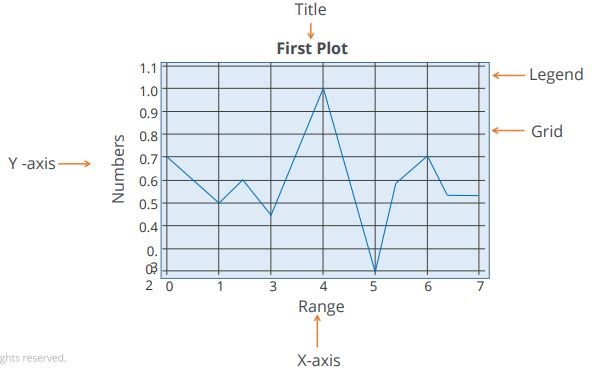We can create a plot using four simple steps.

1. Import the required libraries
2. Define or import the required data set
3. Set the plant parameters
4. Display the created plant

Let's consider the same example plot used earlier. We will follow the steps below to obtain this plot.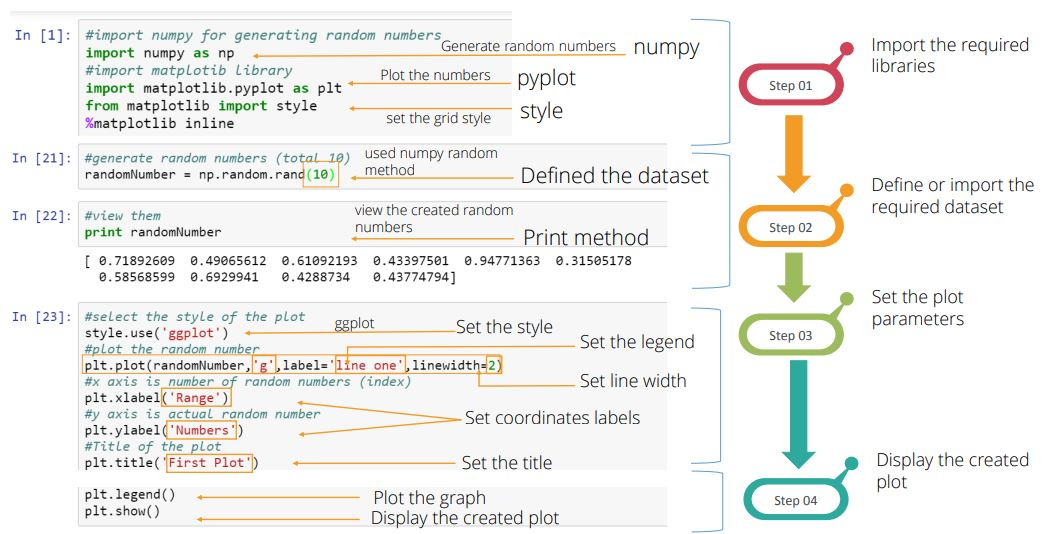The first step is to import the required libraries:

Here we have imported numpy, pyplot, and style from matplotlib

numpy used to generate the random numbers

pyplot is used to plot numbers

style class is used for setting the grid style

matplotlib inline is required to display the plot within the jupyter notebook.

The second step is to define or import the required data set.

Here we have defined the dataset random number using numpy random method. Note that the range is ten. We have used the print method to view the created random numbers

The third step is to set the plot parameters.

In this step, we set the style of the plot, labels of the coordinates, titles of the plot, the legend and the linewidth.

In this example, we have used ggplot as the plot style. The plot method is used to plot the graph against the random numbers. In the plot method the word ‘g’ denotes the plotline color as green, the label denotes the legend label and is named as line one. Also the linewidth=2. Note that we have labeled the x-axis as range and the as labels and set the title as First Plot.

The last step is to display the created plot.

Use the legend method to plot the graph based on the set conditions and the show method to display the created plot.## Line Properties

Let us look at some of the line and Graphics properties below.

1. Alpha
2. Animated

### Plot Graphics

1. Linestyle
2. Linewidth
3. Marker Style

Below table explains the properties and value types of Line Properties.

 Property Value Type alpha float animated [True | False] antialiased or aa [True | False] clip_box a matplotlib.transform.Bbox instance clip_on [True | False] clip_path a Path instance and a Transform instance, a Patch color or c any matplotlib color contains the hit testing function dash_capstyle ['butt' | 'round' | 'projecting'] linestyle or ls [ '-' | '--' | '-.' | ':' | 'steps' | ...] linewidth or lw float value in points marker [ '+' | ',' | '.' | '1' | '2' | '3' | '4' ]

## Creating a 2-D Plot

Consider the following example.

A Nutri world-wide firm wants to know how many people visit its website at a particular time. This analysis helps it control and monitor the website traffic.

This example involves two variables namely users and time. Therefore this is a two dimensional or 2D plot. Take a look at the program that creates a 2D plot.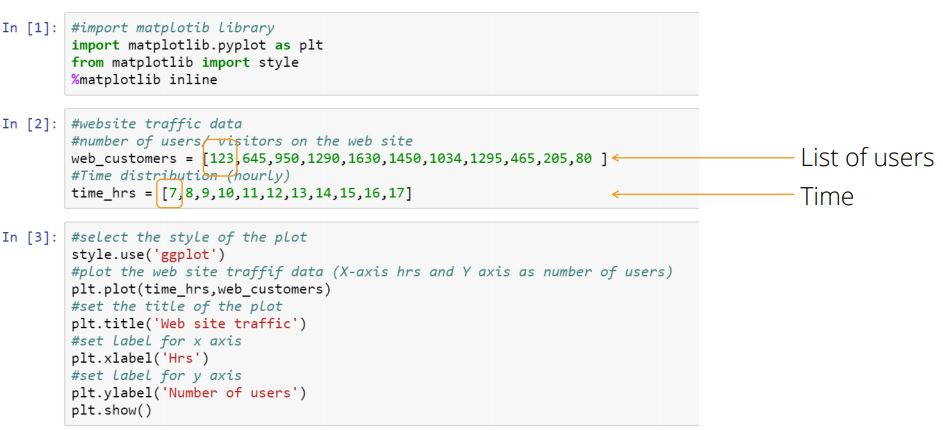Steps

Object web_customers is a list of the number of users and time_hrs indicates the time. From this, we understand that there are 123 customers on the website at 7 AM, 645 customers on the website at 8 AM and so on.

The gg plot is used to set the grid style and the plot method is used to plot the website customers against time. We also need to matplotlib inline to display or view the plot on the jupyter notebook.

The website traffic curve is plotted and the graph is shown on the screen.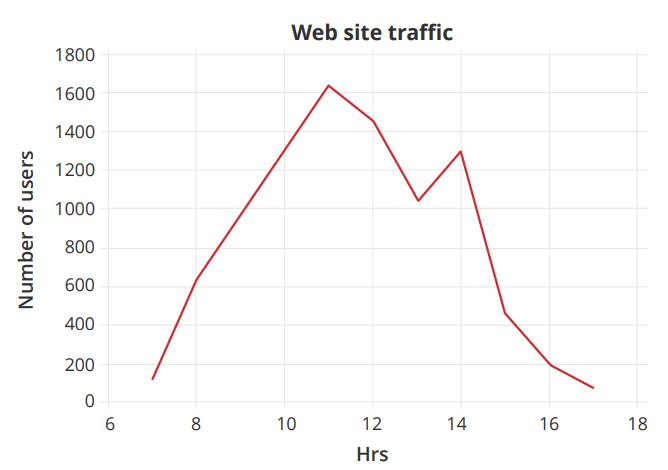It is also possible to change the line style of the plot. To change the line style of the plot as dashed in the plot method, observe the output graph changes to a dashed line. Also, note that the color is defined as blue.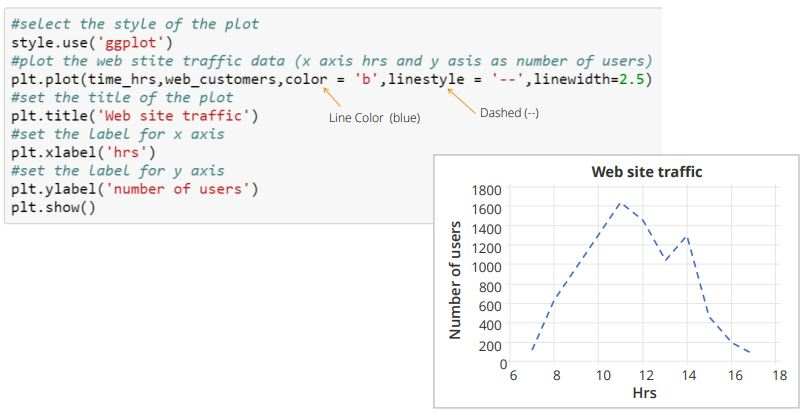Using matplotlib, it is also possible to set the desired axis to interpret the required result. Use the axis method to set the axis. In this example, the x-axis is set to range from 6.5 to 17.5 and the y-axis is set to range from 50 to 2000.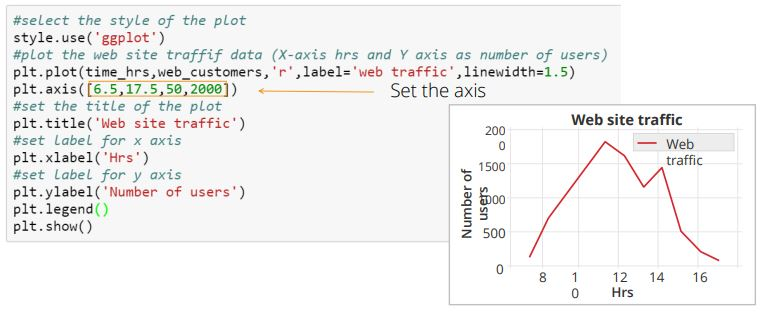### Alpha and Annotation

Let us now understand how to set the transparency of the line and to annotate a plot. Alpha is an attribute which controls the transparency of the line. Lower the alpha value, more transparent than a line.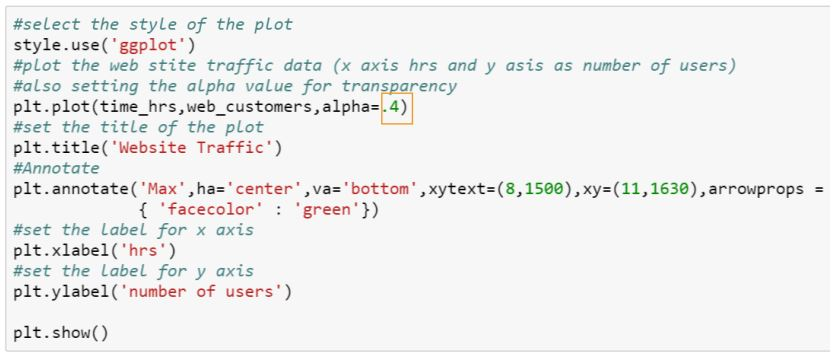Here the Alpha value is defined as 0.4. The Annotate method is used to annotate the graph. The syntax for annotate method is shown in the image above.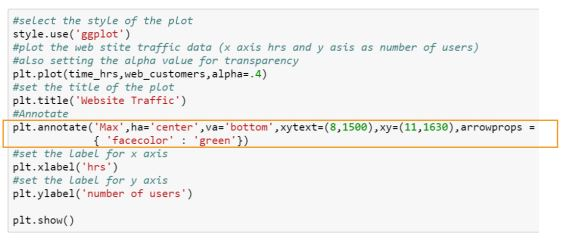The keyword ‘max’ is the attribute that denotes the annotation text.

‘ha’ indicates the horizontal alignment

‘va’ indicates the vertical alignment

‘xytext’ indicates the text position

‘xy’ indicates the arrow position.

The keyword ‘arrowprops’ indicates the properties of the arrow.

In this example, the arrow property is defined as the green color. The output graph is shown below.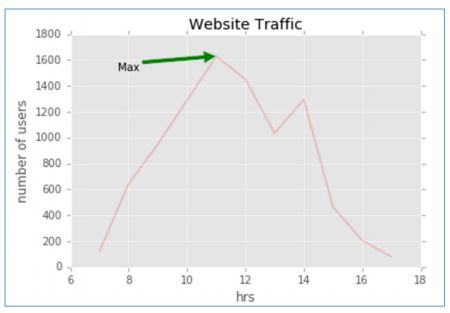## Multiple Plots

So far we've learned how to set linewidth, title, x-axis and y-axis label, the title of the plot, legend, line color and annotate the graph for a single plot. The plot we created for website traffic is for only one day.

Let's now learn how to create multiple plots for three days. We will be using the same example.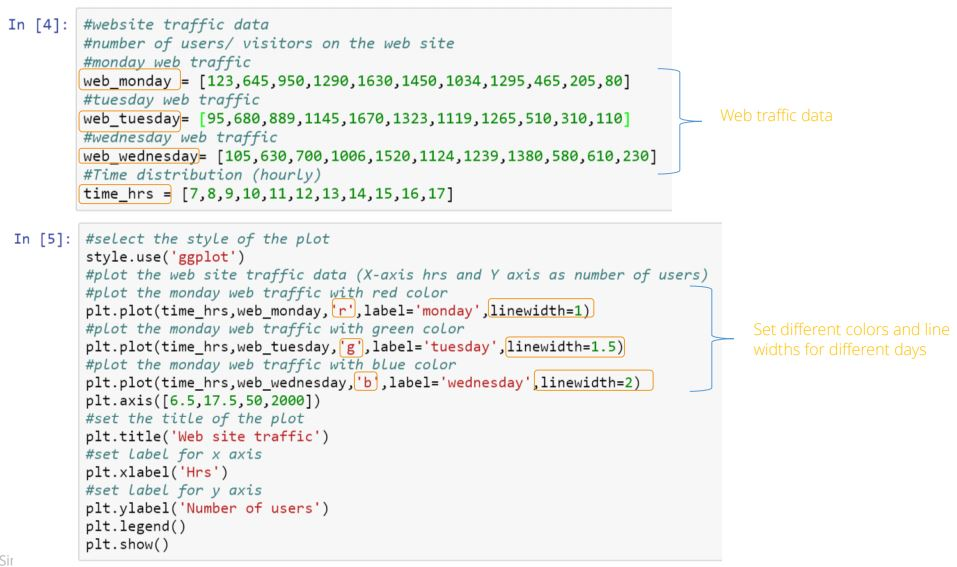The dataset for the number of users for Monday, Tuesday and, Wednesday are defined with respect to its time distribution.

Also, use different color and line for each day to distinguish the plot. In this example, we have used red for Monday, green for Tuesday and blue for Wednesday. The output graph is shown below.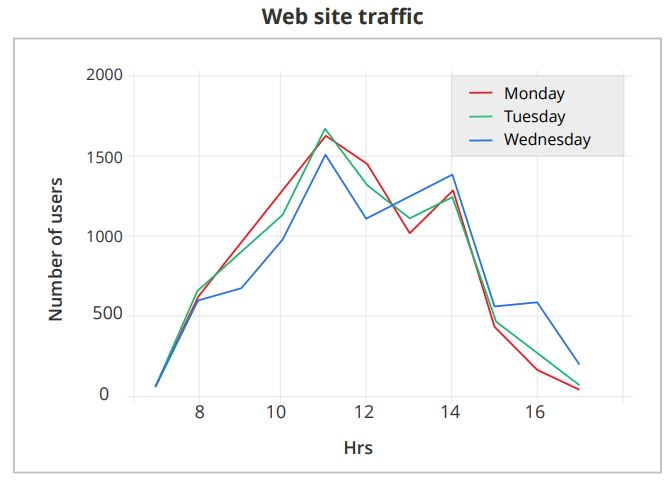### Subplots

A subplot is used to display multiple plots in the same window. With a subplot, we can arrange plots in a regular grid. All we need to do is specify the number of rows, columns, and plot. The syntax for subplot is shown below.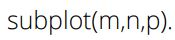It divides the current window into an m by n grid and creates an axis for the subplot in the position specified by p.

For example, Subplot(2,1,2) creates two subplots, which are stacked vertically on a grid. If we want to plot four graphs in one window, then the syntax used should be Subplot(2,1,4).

## Layout and Spacing Adjustments

Layout and spacing adjustment are two important factors to be considered while creating subplots. Use the plt.subplots_adjust method with the parameters hspace and wspace to adjust the distances between the subplot and move them around on the grid.

Two Subplots stacked one on top of the other or vertically split in a single frame and four subplots displayed in a single frame.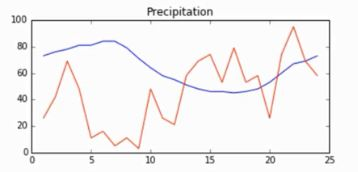Steps

First import matplotlib, pyplot, and style.

Type %matplotlib inline to view the plot in the jupyter notebook.

Define the parameters, such as temperature, wind, humidity, precipitation, data and time data.

Next, create two subplots to be displayed side by side in a given frame for (1,2,1) and (1,2,2). Specify the figure size, subplot_title, the color for time and temperature data which is blue here, and line style and width.

Similarly, specify the color for wind, which is read, it’s line style and width.

We can see the temperature and wind subplot below.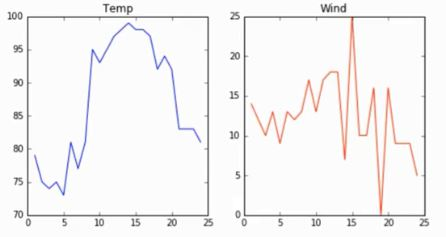Learn more about matplotlib and other Python libraries. Click here!

## Types of Plots

We can create different types of plots using matplotlib:

• Histogram
• Scatter Plot
• Heat Map
• Pie Chart
• Error Bar

### Histograms

Histograms are graphical representations of a probability distribution. In Fact, the histogram is a kind of bar chart. Using matplotlib and its bar chart function, we can create histogram charts.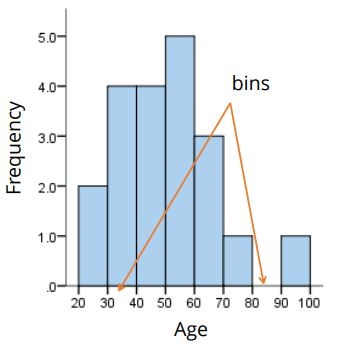A histogram chart has several advantages.

• It displays the number of values within a specified interval.
• It is suitable for large data sets as they can be grouped within the intervals.

### Scatter Plots

A scatter plot is used to graphically display the relationship between the variables. A basic plot can be created using the plot method. However, if we need more control of a plot, it's recommended that we use the scatter() method provided by matplotlib. It has several advantages:

• It shows the correlation between variables
• It is suitable for large data sets
• It is easy to find clusters
• It is possible to represent each piece of data as a point on the plot.

### Heat Maps

A heat map is a better way to visualize two-dimensional data. Using heat maps, we can gain deeper and quicker insight into data than those afforded by other types of plots. It has several advantages:

• It draws attention to the risky-prone area.
• It uses the entire data set to draw bigger and more meaningful insights.
• It's used for cluster analysis and deals with large data sets.

### Pie Chart

Pie charts are typically used to show percentage or proportional data. Usually, the percentage represented by each category is provided next to the corresponding slice of the pie. matplotlib provides the pie method to make pie charts. It has several advantages:

• It summarizes a large data set in visual form.
• It displays the relative proportions of multiple classes of data.
• The size of the circle is made proportional to the total quantity.

### Error Bars

An Error bar used to show the graphical representation of the variability of data. It's used mainly to point out errors and it builds confidence about the data analysis by unleashing the statistical differences between the two groups of data. It has several advantages.

• It shows the variability in data and indicates the errors.
• It depicts the precision of the data analysis.
• It demonstrates how well a function and model are used in the data analysis.
• It defines the underlying data.

## Seaborn

Seaborn is a python visualization library based on matplotlib. It provides a high-level interface for drawing attractive statistical graphics. It was originally developed at Stanford University and is widely used for plotting and visualizing data. There are several advantages:

• It possesses built-in themes for better visualizations.
• It has tools built in statistical functions which reveal hidden patterns in the data set.
• It has functions to visualize matrices of data which become very important when visualizing large data sets.

## Key Takeaways

Let's now quickly recap what we have learned in this tutorial.

• Data visualization is the technique to present the data in a pictorial or graphical format.
• There are three major considerations for data visualization. They are clarity, accuracy, and efficiency.
• The matplotlib is a python 2D plotting library for data visualization and the creation of interactive graphics/ plots.
• A plot is a graphical representation of data which shows the relationship between two variables or the distribution of data.
• Subplots are used to display multiple plots in the same window.
• Seaborn is a Python visualization library based on matplotlib. It provides a high-level interface to draw attractive statistical graphics

## Conclusion

This concludes ‘Data Visualization and Python using matplotlib’ tutorial.

### Find our Data Science with Python Online Classroom training classes in top cities:

Name Date Place
Data Science with Python 3 May -21 May 2021, Weekdays batch Your City View Details
Data Science with Python 14 May -18 Jun 2021, Weekdays batch San Francisco View Details
Data Science with Python 16 May -3 Jun 2021, Weekdays batch Washington View Details
Related Courses
Learner Reviews
Related Articles
• Disclaimer
• PMP, PMI, PMBOK, CAPM, PgMP, PfMP, ACP, PBA, RMP, SP, and OPM3 are registered marks of the Project Management Institute, Inc.

#### Request more information

###### For business
Name*
Email*
Phone Number*
Your Message (Optional)
###### Our consultants will get in touch with you soon.

A Simplilearn representative will get back to you in one business day.

First Name*
Last Name*
Work Email*
Phone Number*
Company*
Job Title*